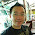## 07 July 2013

### Intrinsic Value - How to Calculate It

In securities investment, especially Shares Investment, there are several methods in defining the actual value of counter. Let me summarize the methods as below:

1. P = D(1 + g) / ( r - g), P = present value, D = dividend, g = dividend growth rate, r = equity requirement rate
2. P = FCFF (1  + g) / ( coc - g), FCFF = firm free cash flow,  coc = cost of capital, g = free cash flow growth rate
3. P = FCFE (1 + g) / ( r - g), FCFE = Equity Free Cash Flow, r = , g = free cash flow growth rate
In short, all the calculation are based on the discounted cash flow from various methods. The value of the shares are relying on the future cash flows. If we are pretty sure of the cash flow, then we can determine lower requirement rate for the counter. The more homework we do on the counter, the better result we could achieve.

#### 2 comments:

1.Hi Jack,
How you come across the valuation by Roger Montgomery the writer of Value.Able. He emphazises on ROE as the key to do valuation. What is your view?

Rgs.

1.ROE is my favorite key finance ratio in fundamental analysis. To break down into 3 parts, you can see ROE = Net Profit Margin * Asset Turnover * Equity Multiplier. For me, I prefer high ROE with <0.5X Debt to Equity Ratio and to be frank, I will look at long term average ROE, such as 5 year ROE to determine the ROE trend during bad / good seasons. I prefer companies that can still invest heavily during bad time so that they can be ready when the market picks up. E.g. You can see now SIA is investing in its luxury flights that currently played by Middle East airplane operators such as Emirates / Qatar Airways. PE Ratio and Dividend Yield as well as Cash Flow are other financial ratios that I will also look into.